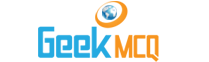# Entry Test MCQ :: Numbers

@ : Home > Math > Numbers

1.  (121x4+3) / (1÷10) ≠?
 A. 3209 B. 4870 C. 2435 x 2 D. 974 x 5 E. 484 x 10+30

2.  Which is closest to 0.5
 A. 1?9 B. 1?3 C. 1?2 D. 4?7 E. 9?14

3.  √(6400/22500) = ?
 A. 101000 B. 5?41 C. 8?15 D. 8?13 E. 16?15

4.  45 / 12+45 / 12+45 / 12 =
 A. 10.9 B. 11.25 C. 12.35 D. 14.32 E. 45 1?4

5.  (10/20) x 10 x (20/20) + (10/20) x 10 x (20/20) = ?
 A. 20?10 B. 100?10 C. 20 D. 100?4 E. 30

6.  Which of the following is true?
 A. 1?3 ? 0.33 B. 45 ?40 ? 5 C. 3450 / 2000=2.25 D. 121 x 11 = 1332 E. 2 ? 4 ? .5

7.  If the sum of five different prime numbers is an even number what is the smallest of the five?
 A. 0 B. 1 C. 2 D. 3 E. 5

8.  If the division of 2 consecutive odd integers gives 0.71 as the result what is the greater of the two?
 A. 3 B. 5 C. 7 D. 9 E. Can not be determined from given information

9.  If an integer R leaves a remainder 5 when it is divided by 8 all are true except.
 A. The number that is 5 less than R is a multiple of 8. B. The number that is 3 more than R is a multiple of 8 C. When divided by 2 R leaves a remainder 1 D. When divided by 4 R leaves a remainder 1 E. When multiplied by 3 R always gives a result which is completely divisible by 2.

10.  If y and z are integers and the sum of yz and z is odd which of the following is true? (I) y and z are even (II) y is odd and z is even (III) y is even and z is odd
 A. I and II B. II only C. II and III D. III only E. I II III